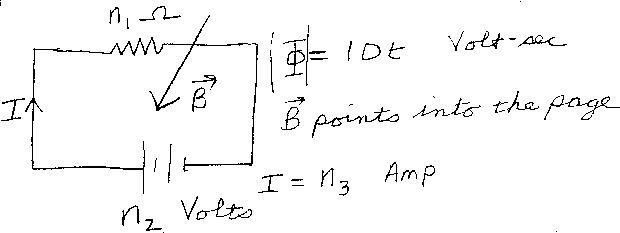Problem C9: In the circuit above the battery has a voltage of n2 Volts. Note that the left side of the battery is positive. The resistor in the circuit has a resistance of n1 Ω. There is a magnetic field that points into the page and is increasing in time. This causes the magnitude of the flux to increase at the rate |Φ| = 10 t Volt-Sec. What is the current in the circuit, if positive current means flowing in the direction of the arrow? That is, if I = n3 Amp, what is n3?n1 = n2 = Input n3:
If you are currently in my class, you can record your grade by entering your name and student ID number (without the leading zeros) below and clicking on "record grade".
 First Name = Last Name = ID = Problem: